# MP Board Class 8th Maths Solutions Chapter 15 Introduction to Graphs Ex 15.3

## MP Board Class 8th Maths Solutions Chapter 15 Introduction to Graphs Ex 15.3

Question 1.
Draw the graphs for the following tables of values, with suitable scales on the axes.
(a) Cost of apples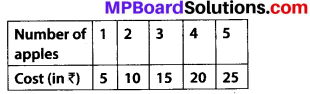(b) The horizontal line x-axis shows the time (in hours) and vertical line y-axis shows the distance (in km).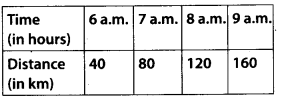(i) How much distance did the car cover during the period 7.30 a.m. to 8 a.m.?
(ii) What was the time when the car had covered a distance of 100 km since it’s start?
(c) interest on deposits for a year.(i) Does the graph pass through the origin?
(ii) Use the graph to find the interest on ₹ 2500 for a year.
(iii) To get an interest of ₹ 280 per year, how much money should be deposited?
Solution:
(a) The horizontal line x-axis shows the number of apples and vertical line y-axis shows the cost (in ₹) of apples.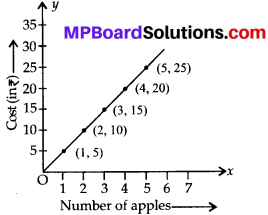(b) The horizontal line x-axis shows the time (in hours) and vertical line y-axis shows the distance (in km).(i) The total distance covered by the car during the period 7.30 a.m. to 8 a.m. is (120 – 100) = 20 km.
(ii) At 7.30 a.m.car had covered a distance of 100 km since its start.
(c) The horizontal line x-axis shows deposit (in ₹) and vertical line y-axis shows simple interest (in ₹)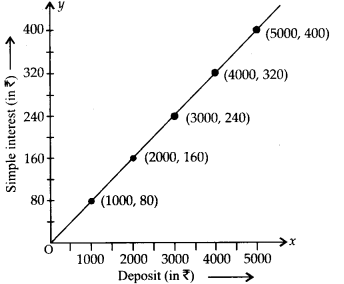(i) Yes, graph passes through the origin.
(ii) Interest on ₹ 2500 is ₹ 200.
(iii) To get an interest of ₹ 280 per year, ₹ 3500 should be deposited.Question 2.
Draw a graph for the followingIs it a linear graph?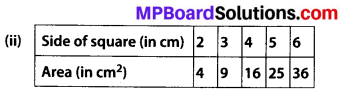Solution:
(i) The horizontal line x-axis shows a side of square (in cm) and the vertical line y-axis shows a perimeter (in cm).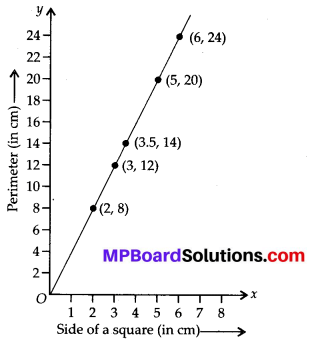Yes, it is a linear graph.
(ii) The horizontal line x-axis shows a side of a square (in cm) and the vertical line y-axis shows an area (in cm2).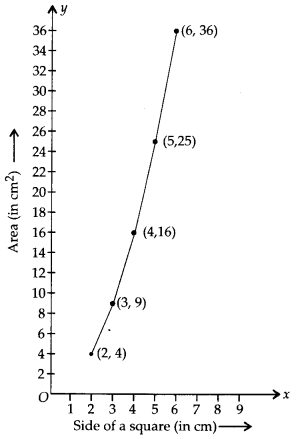No, it is not a linear graph.